Courses

# Geology - 2017 Past Year Paper

## 60 Questions MCQ Test IIT JAM Past Year Papers and Model Test Paper (All Branches) | Geology - 2017 Past Year Paper

Description
This mock test of Geology - 2017 Past Year Paper for IIT JAM helps you for every IIT JAM entrance exam. This contains 60 Multiple Choice Questions for IIT JAM Geology - 2017 Past Year Paper (mcq) to study with solutions a complete question bank. The solved questions answers in this Geology - 2017 Past Year Paper quiz give you a good mix of easy questions and tough questions. IIT JAM students definitely take this Geology - 2017 Past Year Paper exercise for a better result in the exam. You can find other Geology - 2017 Past Year Paper extra questions, long questions & short questions for IIT JAM on EduRev as well by searching above.
QUESTION: 1

Solution:
QUESTION: 2

Solution:
QUESTION: 3

### En- echelon sigmoidal ‘gash’ veins indicate

Solution:
QUESTION: 4

Which one of the following primary sedimentary structures is NOT used for palaeocurrent analysis?

Solution:
QUESTION: 5

The age of the Patcham Formation is

Solution:
QUESTION: 6

Rivers that receive water from groundwater seepage are termed as

Solution:
QUESTION: 7

Conservative plate boundary is represented by

Solution:
QUESTION: 8

Which one of the following prismatic crystal forms belongs to the hexagonal crystal system ?

Solution:
QUESTION: 9

The characteristic rock of contact metamorphism is

Solution:
QUESTION: 10

The volcanic equivalent of nepheline syenite is

Solution:
QUESTION: 11

Identify the correct match between mineral- ore and its physical property.

Solution:
QUESTION: 12

Match the mineral deposits in Gourp I with their Indian occurrences in Group II.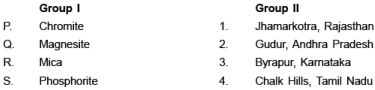Solution:
QUESTION: 13

A helically coiled ammonite Turrilites is differentiated from externally resembling Gastropoda Turritella by

Solution:
QUESTION: 14

The facial suture of trilobites running through the genal angle is known as

Solution:
QUESTION: 15

Which one of the following statements is correct for Class 1B (Parallel) folds ?

Solution:
QUESTION: 16

In the given map, the X- Y surface has the same orientation as in the Palaeozoic sequence, XY represents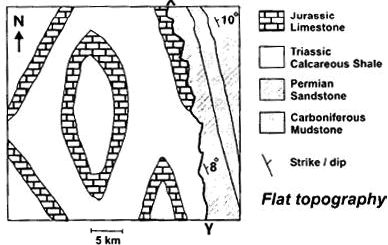Solution:
QUESTION: 17

Match the sedimentary features in Group I with the corresponding sedimentary environments of their formation in Group II.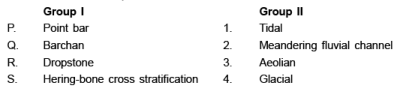Solution:
QUESTION: 18

Which one of the following lithostratigraphic units id of Phanerozoic Eon ?

Solution:
QUESTION: 19

Match the geological processes (Group I) with their examples in Indian stratigraphy (Group II).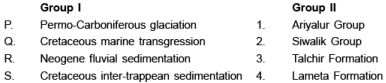Solution:
QUESTION: 20

Check dams are constructed in association with main dam in the

Solution:
QUESTION: 21

A geological formation neither containing not transmitting water is termed as

Solution:
QUESTION: 22

Which one of the following sequences of silicate structures indicates an increasing degree of sharing of corners of (SiO4)4– tetrahedra ?

Solution:
QUESTION: 23

Match the igneous bodies in Group I with their ages in Group II.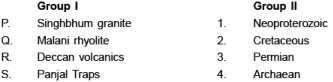Solution:
QUESTION: 24

The tubefeet in echinoids emerge through

Solution:
QUESTION: 25

A mineral with a point group symmetry 2/m

Solution:
QUESTION: 26

The progressive metamorphic isograd that explains Barrovian metamorphism in pelite is

Solution:
QUESTION: 27

In metabasic rocks, plagioclase is not stable in

Solution:
QUESTION: 28

A sandstone has <5% matrix. The recalculated modal compositions of feldspar, quartz and rock fragments are 45%, 35%, 20%, respectively. The sandstone is classified as

Solution:
QUESTION: 29

Match the earth layers (Group I) with corresponding approximate thicknesses (Group II).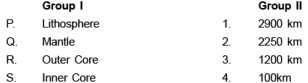Solution:
QUESTION: 30

Pressure (1 GPa = 10 kbar) and temperature at the centre of the Earth are estimated to be

Solution:
*Multiple options can be correct
QUESTION: 31

Choose the landsform(s) resulting from glacial erosion.

Solution:
*Multiple options can be correct
QUESTION: 32

Choose the correct combination(s) of type of dentition of Bivalvia and the corresponding representative genus.

Solution:
*Multiple options can be correct
QUESTION: 33

Shown below is an isobaric binary temperature- composition phase diagram in the system X- Y with complete miscibility between X and Y.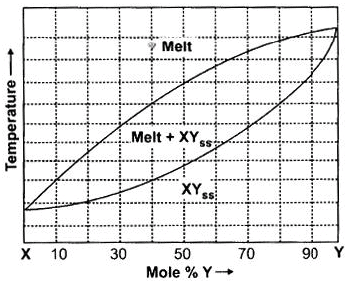W hich of the following statements is/are correct for crystallization of a starting melt of composition Z60 Y40 (the dot in the diagram) ?

Solution:
*Multiple options can be correct
QUESTION: 34

Choose the correct combination(s) of textural features of magmatic rocks with corresponding petrological processes from the following.

Solution:
*Multiple options can be correct
QUESTION: 35

Which of the following statements is/are NOT correct ?

Solution:
*Multiple options can be correct
QUESTION: 36

In an outcrop we find that the bedding planes are vertical and cleavage surfaces are horizontal.Which of the following fold types is/are inferred from this observation ?

Solution:
*Multiple options can be correct
QUESTION: 37

Which of the following stratigraphic unit(s) is/are coal/lignite bearing ?

Solution:
*Multiple options can be correct
QUESTION: 38

The Toposheet No(s). immediately adjacent to Toposheet No. 55J/8 is/are

Solution:
*Multiple options can be correct
QUESTION: 39

Which of the following is/are NOT true for texturally immature sandstone ?

Solution:
*Multiple options can be correct
QUESTION: 40

P and S waves originate at earthquake focus and travel through the earth. Which of the following statements for these waves is/are correct ?

Solution:
*Answer can only contain numeric values
QUESTION: 41

An object is spoted at S60°E front bearing from the observer. If the position is interchanged, the front bearing value in degree from North (measured clockwise) is_____.

Solution:
*Answer can only contain numeric values
QUESTION: 42

The mole % of forsterite component in olivine with chemical formula Mg1.8 Fe0.2 SiO4 is _____.

Solution:
*Answer can only contain numeric values
QUESTION: 43

The Weiss symbol of a crystal face is 4a: 2b: c. The value of h in the corresponding Miller Index (hkl) is _____.

Solution:
*Answer can only contain numeric values
QUESTION: 44

In a mineral with chemical formula AT4 O8, the ionic radii of A and O are 1.12 Å and 1.40 Å, respectively. The co- ordination number of cation A is ____.

Solution:
*Answer can only contain numeric values
QUESTION: 45

Aluminium (Al) can occur in both tetrahedral and octahedral co- ordinations in silicates. The amount of octahedral Al in a pyroxene crystal of composition MG1.4 Fe0.4 Al0.4 Si1.8 O6 is ____ (give answer in one decimal place).

Solution:
*Answer can only contain numeric values
QUESTION: 46

The birefringence of a mineral of thickness 30 mm and retardation 0.27 mm is _____ (give answer in three decimal places).

Solution:
*Answer can only contain numeric values
QUESTION: 47

Two limbs of a vertical chevron fold strike S70°E and N55°E. The value of the interlimb angle of the fold is ____(degree).

Solution:
*Answer can only contain numeric values
QUESTION: 48

The schematic map given below shows intersecting strike lines of the same lithological contact.
In the map, AB and CD are 5cm and 3.5cm, respectively. The scale of the map is 1 cm = 100m.
The plunge of the fold axis is ____ degrees (give answer in one decimal place).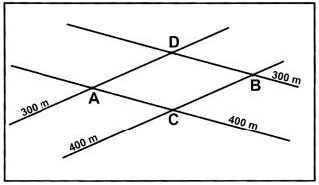Solution:
*Answer can only contain numeric values
QUESTION: 49

The core- rim compositions of a normally zoned plagioclase crystal are as follows: Core: Ca0.6 Nax Al1.6 Si2.4 O8 Rim: Ca0.4 Nay Al1.4 Si2.6 O8 The amount of increase of Na atom from core to rim per formula unit of plagioclase is _____ (given answer in one decimal place).

Solution:
*Answer can only contain numeric values
QUESTION: 50

Considering garnet chemical formula in 12 oxygen basis, the number of Mg cations in a garnet of chemical composition P (as shown in the figure) is ____ (give answer in two decimal places).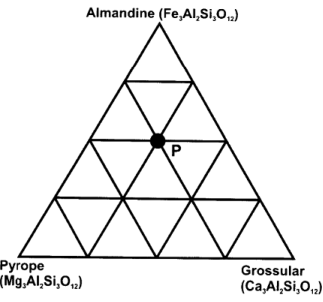Solution:
*Answer can only contain numeric values
QUESTION: 51

A fault surface in an outcrop has slickenside lineation whose pitch is 30°. The horizontal slip on the fault is 1.25 m, as determined from displaced vein. The net slip on the fault is ____ meter (give answer in two decimal places).

Solution:
*Answer can only contain numeric values
QUESTION: 52

In an outcrop, we find a Belemnite fossil broken into five rectangular pieces (boudins) of equal size. Long dimension of each boudin is 1.35 cm. Gap between adjacent boudins in all cases is 0.25 cm. Note that the long dimensions of boudins are perfectly aligned. The % elongation is _____ (give answer in one decimal place).

Solution:
*Answer can only contain numeric values
QUESTION: 53

A horizontal cylindrical ore body (diameter = 20m, length = 200 m) has 5% metal content and density of 3500 kg/m3. The reserve of the ore body is ____ million ton(s) (give answer in two decimal places).

Solution:
*Answer can only contain numeric values
QUESTION: 54

A drainage basin of fourth order covers an area of 40 sq. km. Within the basin, total length of 1st order drainage is 12.5 km, 2nd order drainage is 8.8 km, 3rd order drainage is 4.7 km and 4th order drainage is 4.0 km. The drainage density of the basin is ___ km–1 (give answer in two decimal places).

Solution:
*Answer can only contain numeric values
QUESTION: 55

Age of granitic rocks can be determined using Rb- Sr whole rock radioactive dating method and the following age equation,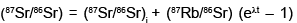For a suite of representative co- magmatic granitic rocks, the Rb- Sr whole rock isochron plot and relevant data are shown in the diagram. The age of granite is calculated at ____ Ga (1Ga = 109 yrs, give answer in one decimal place).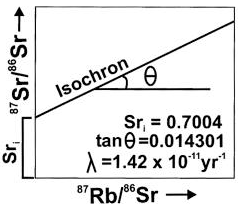Solution:
*Answer can only contain numeric values
QUESTION: 56

Consider a granulite facies metamorphic rock with peak metamorphic condition at 9 kbar, 850°C.
Assume a single layer crust of ρ = 3000 kg/m3 and g = 10m/sec2 during metamorphism. The depth of burial during peak metamorphism is ____ km. 1 Pascal = 1 kg/m/sec2 and 1 bar = 105 Pascals.

Solution:
*Answer can only contain numeric values
QUESTION: 57

Consider four minerals P, Q, R and S in a three component chemical system (A- B- C) as shown in the figure. For a crossing tie- line relationship, the variance (degree of freedom) of the equilibrium mineral assemblage at X is ____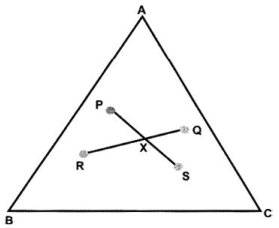Solution:
*Answer can only contain numeric values
QUESTION: 58

The refractive indices of four minerals (P, Q, R, S) are as follows:

P(α = 1.712, β  = 1.721, λ  = 1.727),

Q(ε  = 1.553, ω  = 1.544),

R(α  = 1.664, β  = 1.672, λ  = 1.694) and

S(ω  = 1.658, ε  = 1.486)

The value of maximum briefringence among all the minerals is ____ (give answer in two decimal places).

Solution:
*Answer can only contain numeric values
QUESTION: 59

In a sedimentary succession shown in the figure, the last occurrence of the fossil species Q (dated 50 Ma) and the first occurrence of the fossil species R (dated 30 Ma) are recorded at Y and X, respectively. The estimated rate of sedimentation is _____ m/million yrs (assume constant rate of sedimentation).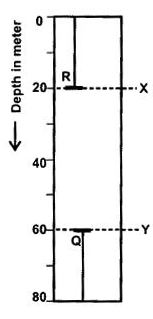Solution:
*Answer can only contain numeric values
QUESTION: 60

The top surface of a coal seam is exposed at 150 m contour level on a hill top at location A.
The same surface of the seam is also exposed on a river bed at location B at the 50 m contour level. The aerial distance A- B is 1 km. The amount of dip of the coal seam along A- B is ___(degree). Give answer in one decimal place.

Solution: